Mechanics

# gravitational potential energy

Gravitational potential energy is a scalar quantity , measured in joules , which measures the amount of energy attributed to a body of a certain mass that is at a certain height in relation to the ground. The formula used to calculate it is obtained from Isaac Newton’s law of universal gravitation , so it is part of the equations used to describe all types of gravitational interactions.

## What is gravitational potential energy?

Gravitational potential energy is a form of energy associated with the height at which a body is in a region of non-zero gravitational field . The presence of a gravitational field assigns gravitational potential energy to every body that has mass and that has some height in relation to the mass that produces gravity in the region.

It can be calculated using a very simple formula , determined by the product of the quantities mass, acceleration due to gravity and height, as shown below: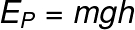m – body mass (kg)

g – acceleration due to gravity (m/s²)

h – height (m)

The unit of measurement for gravitational potential energy, according to the International System of Units , is the joule (J) .

→ Example

We want to calculate the intensity of gravitational potential energy on a 1 kg body that is at a height of 10 meters, in a region of the Earth where the acceleration due to gravity is approximately 9.8 m/s²: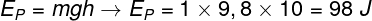## Formula for gravitational potential energy

The formula used to calculate gravitational potential energy is derived from the definition of work , that is, gravitational potential energy is equivalent to the work done to lift an object from the ground to a height h. Furthermore, the most fundamental definition of gravitational potential energy comes from the law of universal gravitation, developed by the English physicist Isaac Newton .

According to the law of universal gravitation, the force of weight produced by gravity is exclusively attractive and acts on an imaginary line that passes through the centers of mass of two bodies of masses M and m. It is also inversely proportional to the inverse square of the distance d that separates these two bodies. It can be expressed this way: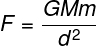G – constant of universal gravitation (6.67408.10 -11 N. kg²/m²)

Still according to the law shown above, it is possible to obtain an expression to calculate the gravitational potential energy, namely: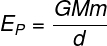## elastic potential energy

Elastic potential energy is a type of potential energy , just like gravitational potential energy. This indicates that this is a form of energy that can be stored and later transformed into other forms of energy.

Elastic potential energy originates from the deformation of bodies that tend to return to their original shape after being deformed. In this sense, this form of energy depends on a characteristic called the elastic constant , as well as on the elongation or deformation of the body. The formula used to calculate elastic potential energy is as follows: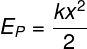k – spring constant (N/m)

x – deformation of the body (m)

## Solved exercises on gravitational potential energy

question 1 — Calculate the magnitude of gravitational potential energy that a 5 kg body has when placed at a height of 10 meters above the ground. Consider local gravity to be g = 9.8 m/s².

a) 180 J

b) 490 J

c) 250 J

d) 150 I

Resolution:

To make the calculation, it is necessary to multiply the quantities presented: mass, gravity and height.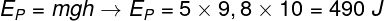Based on the calculation, we find that the gravitational potential energy stored in this body is equal to 490 J and that therefore the correct alternative is the letter B .

Question 2 — Calculate the height required for a 2.5 kg body to present a gravitational potential energy of 500 J. Consider local gravity equal to 10 m/s².

a) 50 m

b) 30 m

c) 20 m

d) 15 m

Resolution:

To calculate the height at which the body is, we need to use the formula for gravitational potential energy.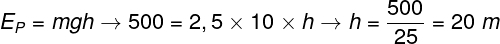Based on the result obtained, we determined that the correct alternative is the letter C.